Next: Spin-orbit coupling and fine-structure Up: Angular momentum and its Previous: Normal Zeeman effect

#### Electron spin

In the year 1921, Stern and Gerlach performed a famous experiment which is now known by their names, in which a beam of silver atoms having net zero orbital angular momentum was made to pass through an inhomogeneous magnetic field. It was observed that the beam was split into two beamlets due to a deflecting force on the magnetic moments present in the atoms. This magnetic moment could not have originated from the orbital angular momentum. This led Uhlenbeck and Goudsmit in 1925 to introduce the concept of another intrinsic angular momentum of the atom due to electron-spin. This was called the spin angular momentum (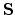) and its resultant magnetic moment () could successfully account for the splitting of the beam of Silver atoms in Stern-Gerlach experiment.

Just as (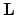) is quantized so is ():

|| =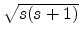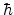and Sz = msis the spin angular momentum of the electron (or the atom) and Sz is its z-component. s is called the spin quantum number has a single value of 1/2 and ms, the spin magnetic quantum number ms has two values +1/2 and -1/2.

Each Ag atom in Stern-Gerlach experiment has a spin-angular momentumdue to the spin of a single electron, since the ground state electronic configuration of Ag atom is 4d105s1, i.e. a single unpaired electron resides outside a closed shell.

Since Sz = ms=(1/2), the Ag beam splits into two beamlets in a vertical plane through the z-axis.

The electron-spin was investigated more formally by Dirac using relativistic quantum mechanics (an amalgamation of quantum mechanics and special theory of relativity). Dirac used the same postulates of Schrodinger's quantum mechanics but used the relativistic expression for the total energy. He went on to prove that an electron must have an intrinsic spin angular momentum and an associated spin magnetic moment. It may be pointed out that the picture of the electron spinning about its axis is not quite correct. Instead it would be better to think of the electron spin as having a magnitude of (1/2)and enjoying the same status as other fundamental constants like charge and mass of the electron.

It was also shown by Dirac that the electron magnetic moment () and electron spin angular momentum () are related as follows: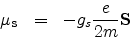where gs is called the 'spin g-factor' having a value of approximately 2. It is also equal to the gyromagnetic ratio which is the ratio of the spin to orbital g- factors. The 'orbital g-factor' has a value of 1.Next: Spin-orbit coupling and fine-structure Up: Angular momentum and its Previous: Normal Zeeman effect
Abhijit Poddar
2007-09-27